# How to Convert Between Minutes and Hours

How to Convert Between Minutes and HoursThere are 60 minutes in 1 hour.

To convert minutes to hours, divide by 60.

To convert hours to minutes, multiply by 60.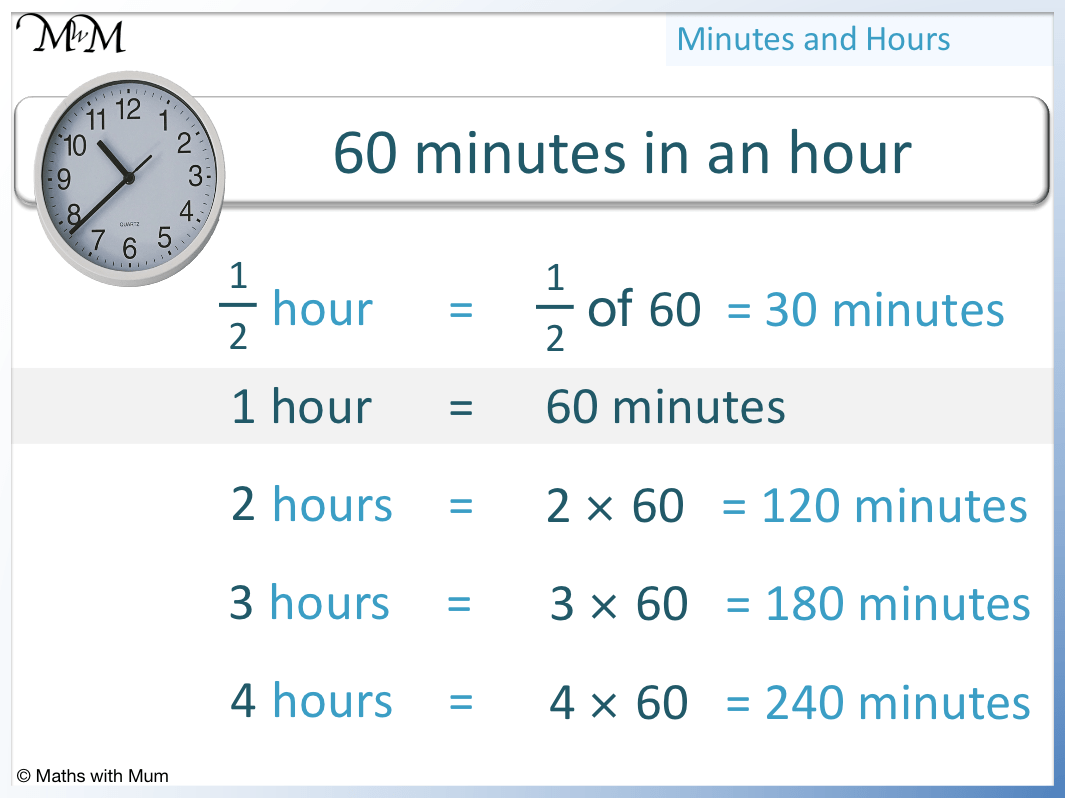• To convert hours to minutes, multiply by 60.
• 1 hour = 60 minutes.
• 2 hours = 120 minutes.
• 3 hours = 180 minutes.
• 4 hours = 240 minutes.
• 1/2 of an hour = 30 minutes.
• 1/4 of an hour = 15 minutes.• 2 × 60 = 120, therefore there are 120 minutes in 2 hours.
• 1/2 of 60 is 30, therefore there are 30 minutes in half an hour.
• Therefore the number of minutes in 2 and a half hours is 120 + 30 = 150 minutes.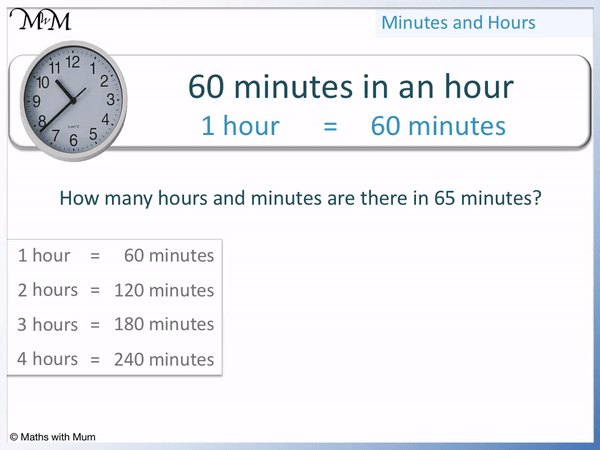• To convert minutes to hours, we can divide by 60
• Alternatively, we can subtract lots of 60.
• Each lot of 60 minutes that can be subtracted equals one hour.
• 65 – 60 = 1 remainder 5.
• Therefore 65 minutes = 1 hour 5 minutes.Supporting Lessons

# Converting Units of Time

## How to Convert Hours to Minutes

To convert hours to minutes, multiply the number of hours by 60. For example, 3 hours = 180 minutes since 3 × 60 = 180.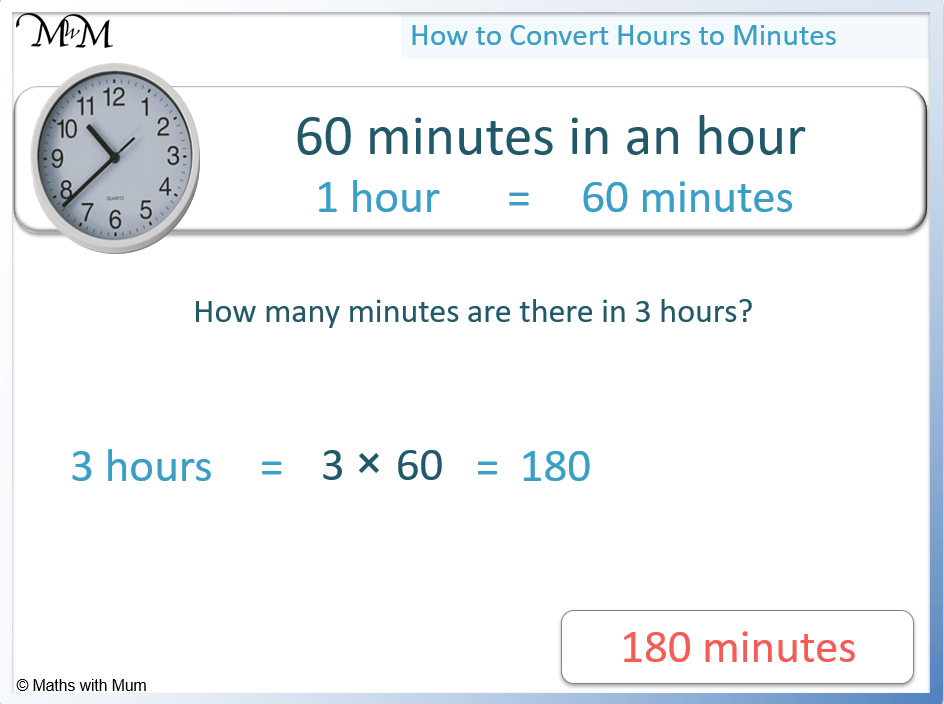The formula to convert hours to minutes is: minutes = hours × 60.For example, convert 4.5 hours to minutes.

4.5 × 60 = 270, therefore there are 270 minutes in 4.5 hours.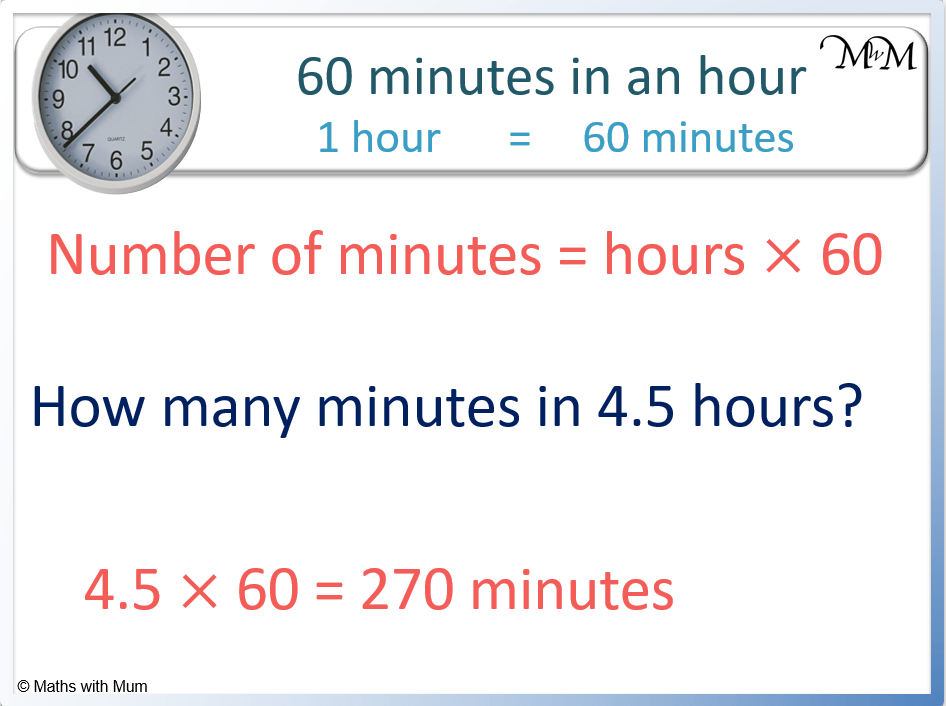The table below shows some common conversions of hours to minutes.

Hours Minutes
1/60 of an hour 1 minute
1/30 of an hour 2 minutes
1/20 of an hour 3 minutes
1/10 of an hour 6 minutes
1/5 of an hour 12 minutes
1/4 of an hour 15 minutes
1/3 of an hour 20 minutes
1/2 of an hour 30 minutes
1 hour 60 minutes
1 1/2 hours 90 minutes
2 hours 120 minutes
2 1/2 hours 150 minutes
3 hours 180 minutes
3 1/2 hours 210 minutes
4 hours 240 minutes
4 1/2 hours 270 minutes
5 hours 300 minutes

We can combine values from this table to find the number of minutes in a given number of hours.

For example, convert two and a half hours to minutes.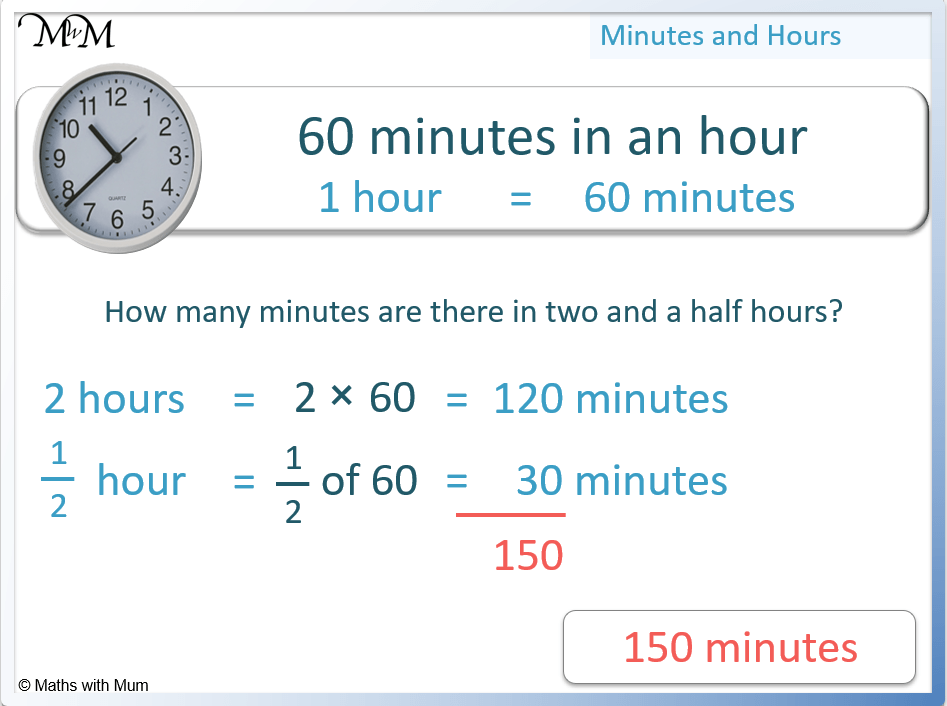There are 120 minutes in 2 hours.

There are 30 minutes in half an hour.

Therefore there are 150 minutes in 2 and a half hours.

For example, find the number of minutes in 3 hours 40 minutes.

There are 180 minutes in 3 hours.

180 + 40 = 220 minutes and so, there are 220 minutes in 3 hours 40 minutes.## How to Convert Minutes to Hours

To convert minutes to hours, divide the number of minutes by 60. For example, 120 minutes = 2 hours because 120 ÷ 60 = 2.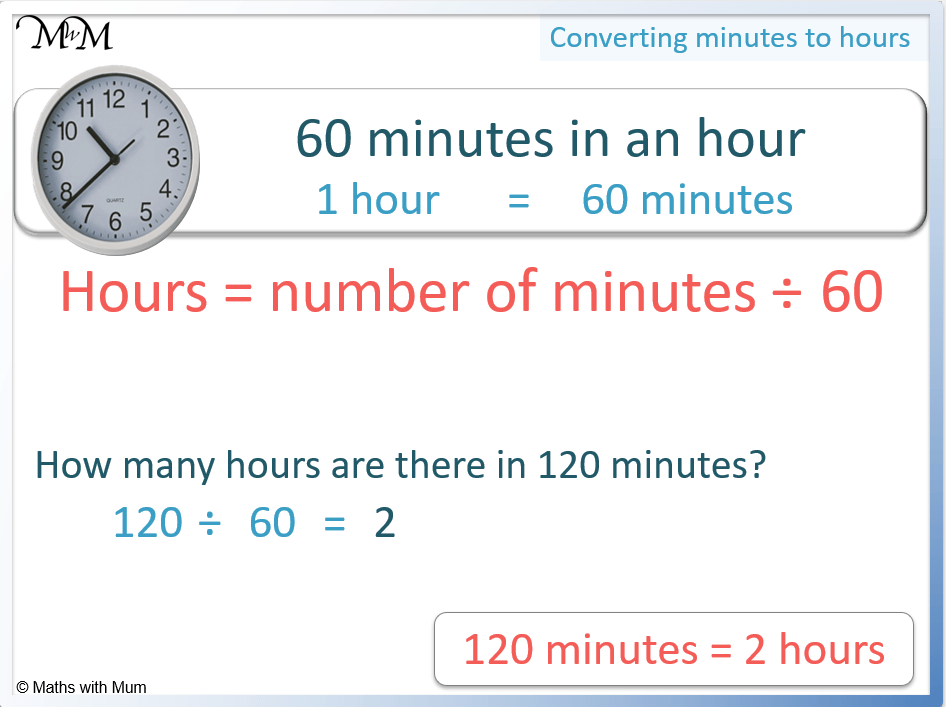The formula to convert minutes to hours is: Hours = Number of minutes ÷ 60.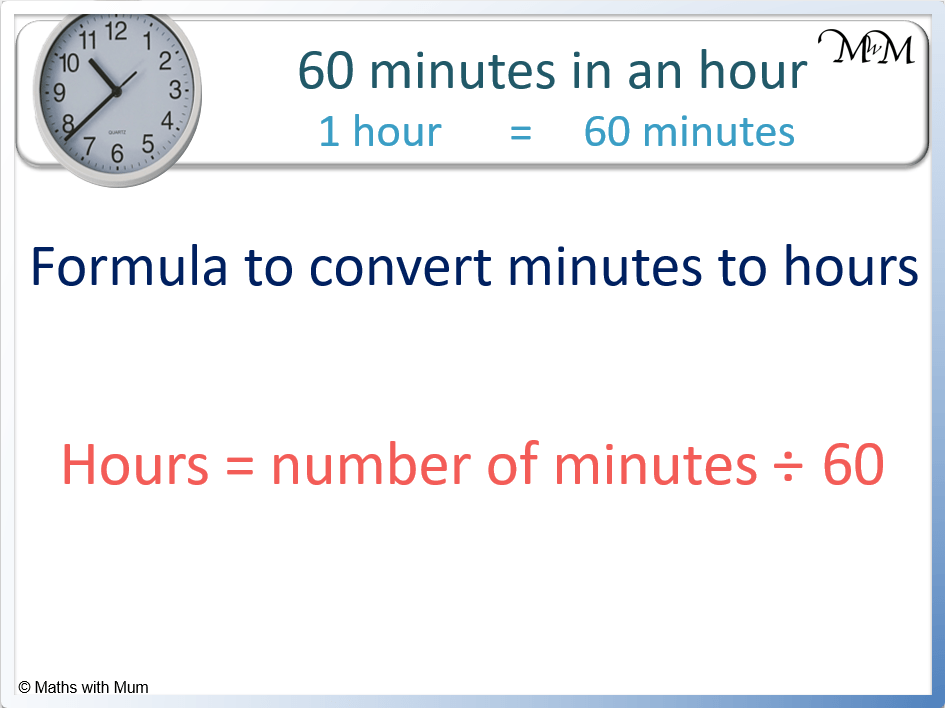For example, convert 240 minutes to hours.

240 ÷ 60 = 4 and so, there are 4 hours in 240 minutes.The following table shows the conversion of minutes to hours.

Number of Minutes Hours
15 minutes 0.25 (one quarter) of an hour
20 minutes 0.33 (one third) of an hour
30 minutes 0.5 (one half) of an hour
45 minutes 0.75 (3 quarters) of an hour
60 minutes 1 hour
90 minutes 1.5 (one and a half) hours
120 minutes 2 hours
150 minutes 2.5 (2 and a half) hours
180 minutes 3 hours
210 minutes 3.5 (3 and a half) hours
240 minutes 4 hours
270 minutes 4.5 (4 and a half) hours
300 minutes 5 hours

## How to Convert a Time to Hours and Minutes

To convert a time in minutes to hours and minutes:

1. Divide the number of minutes by 60.
2. The number of hours is the integer part of the result.
3. The number of minutes is found by multiplying the decimal part of the number by 60.

For example, convert 150 minutes to hours and minutes.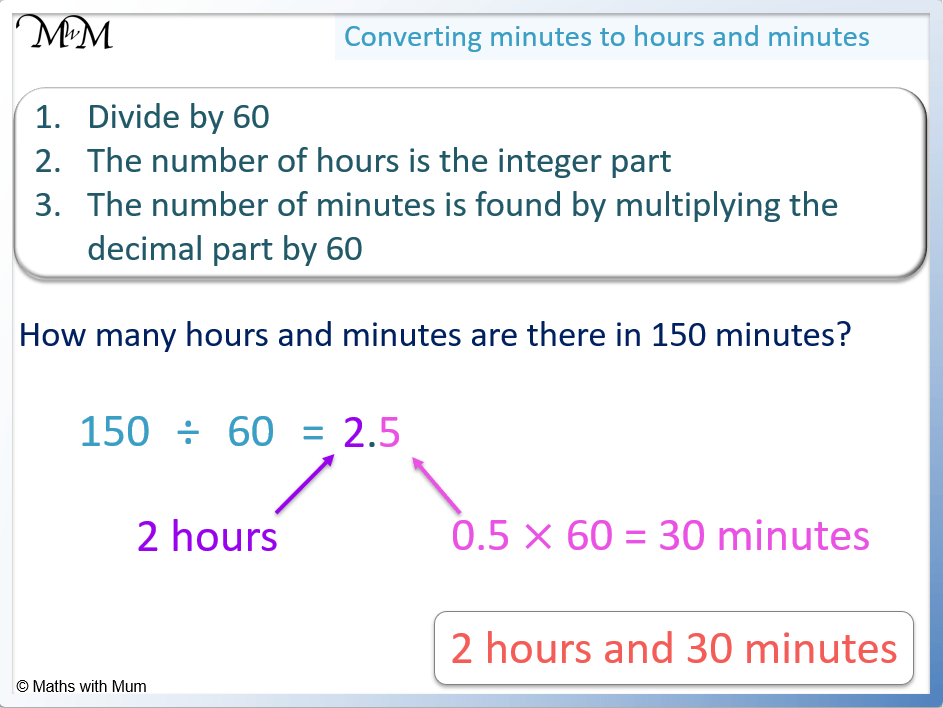Step 1. Divide the number of minutes by 60

150 ÷ 60 = 2.5.

This number is made up of 2 + 0.5.

Step 2. The number of hours is the integer part of the result

The integer part of the result is the part of the number in front of the decimal point.

Before the decimal point is a 2. Therefore the number of hours is 2.

Step 3. The number of minutes is found by multiplying the decimal part of the number by 60

The decimal part of the number is 0.5.

0.5 × 60 = 30 and so there are 30 minutes.

150 minutes = 2 hours and 30 minutes.

For example, convert 340 minutes to hours and minutes.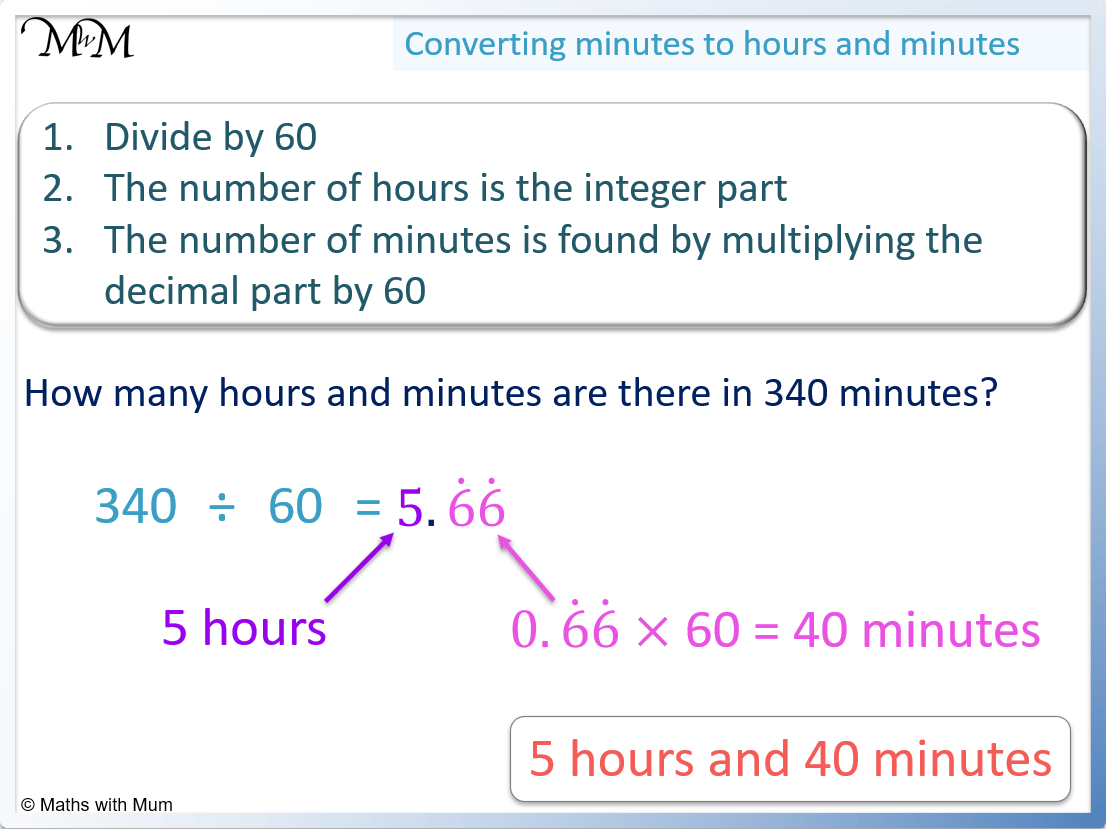Step 1. Divide the number of minutes by 60

340 ÷ 60 = 5.66.

This is made up of 5 + 0.66.

Step 2. The number of hours is the integer part of the result

The integer part of the result is 5. There are 5 hours.

Step 3. The number of minutes is found by multiplying the decimal part of the number by 60

The decimal part of 5.66 is 0.66.

0.66 × 60 = 40 and so, there are 40 minutes.

340 minutes = 5 hours and 40 minutes.

#### Alternative Method for Converting a Time to Hours and Minutes

To convert a time in minutes to hours and minutes, subtract multiples of 60 from the number. The number of times 60 can be subtracted is the number of hours and the remainder is the number of minutes.

For example, convert 175 minutes into hours and minutes.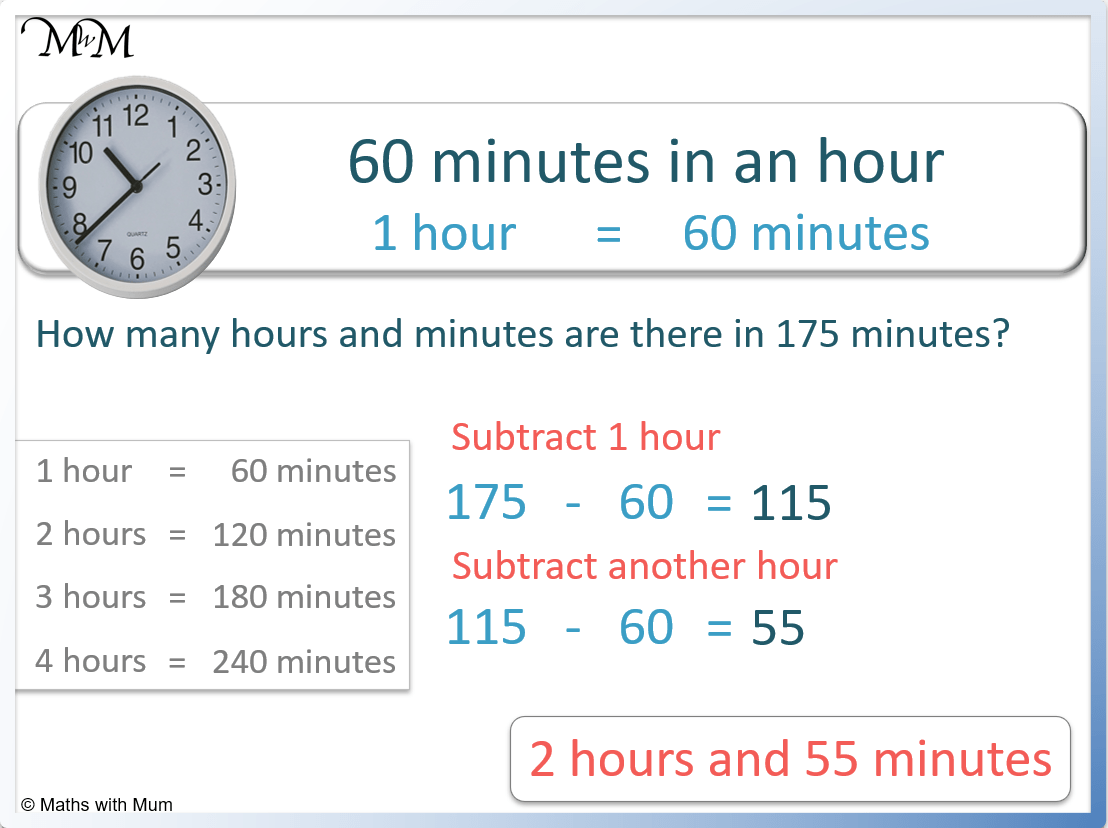175 – 60 = 115.

115 – 60 = 55.

Therefore we can subtract two lots of 60, which is 2 hours.

The remainder is 55 minutes.

175 minutes = 2 hours 55 minutes.

For example, convert 225 minutes into hours and minutes.

Subtract one hour: 225 – 60 = 165.

Subtract a second hour: 165 – 60 = 105.

Subtract a third hour: 105 – 60 = 45.

225 minutes = 3 hours and 45 minutes.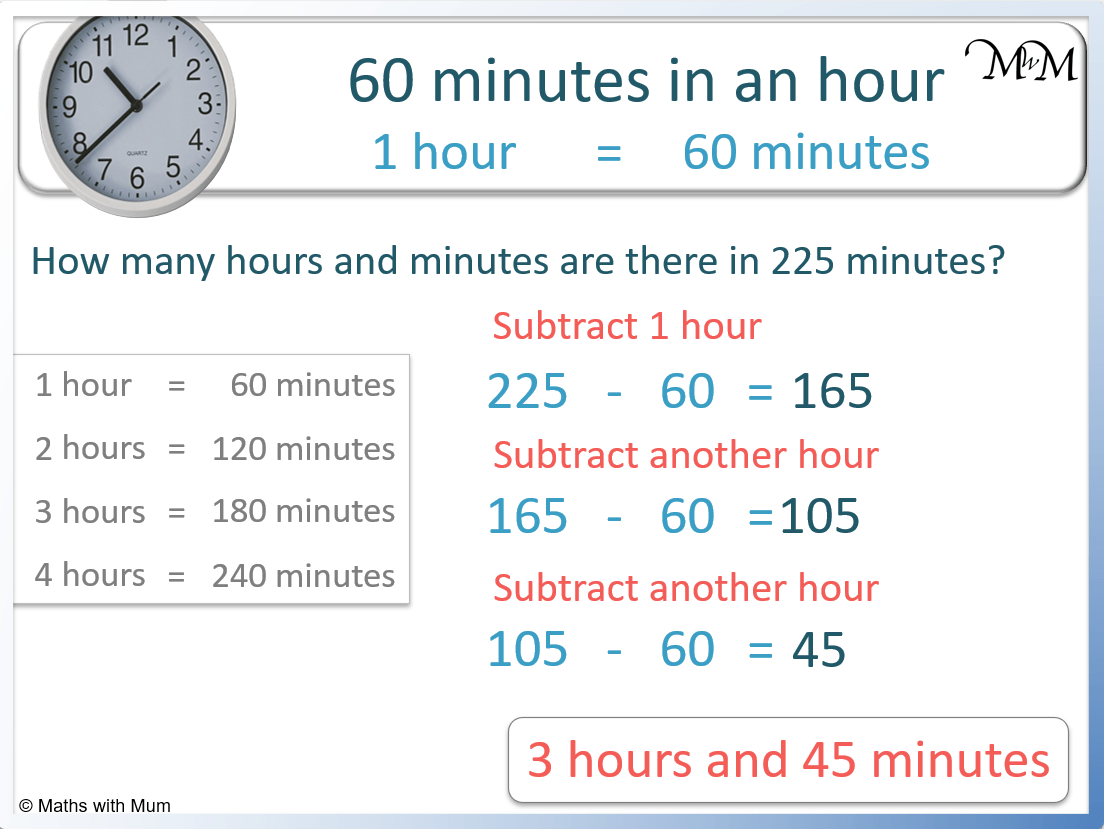## Minutes to Hours Conversion Chart

Minutes Hours Hours and Minutes
1 min 0.0166 hr 0 hr 1 min
2 min 0.033 hr 0 hr 2 min
3 min 0.05 hr 0 hr 3 min
4 min 0.066 hr 0 hr 4 min
5 min 0.0833 hr 0 hr 5 min
6 min 0.1 hr 0 hr 6 min
7 min 0.1166 hr 0 hr 7 min
8 min 0.133 hr 0 hr 8 min
9 min 0.15 hr 0 hr 9 min
10 min 0.166 hr 0 hr 10 min
15 min 0.25 hr 0 hr 15 min
20 min 0.33 hr 0 hr 20 min
30 min 0.5 hr 0 hr 30 min
40 min 0.66 hr 0 hr 45 min
45 min 0.75 hr 0 hr 45 min
50 min 0.833 hr 0 hr 50 min
60 min 1 hr 1 hr 0 min
70 min 1.166 hr 1 hr 10 min
80 min 1.33 hr 1 hr 20 min
90 min 1.5 hr 1 hr 30 min
100 min 1.66 hr 1 hr 40 min
120 min 2 hr 2 hr 20 min
180 min 3 hr 3 hr 0 min
200 min 3.33 hr 3 hr 20 min
240 min 4 hr 4 hr 0 min
300 min 5 hr 5 hr 0 min
400 min 6.66 hr 6 hr 40 min
500 min 8.33 hr 8 hr 20 min
600 min 10 hr 10 hr 0 min
700 min 11.66 hr 11 hr 40 min
800 min 13.33 hr 13 hr 20 min
900 min 15 hr 15 hr 0 min
1000 min 16.66 hr 16 hr 40 min
1440 min 24 hr 24 hr 0 min
2880 min 48 hr 48 hr 0 min
3000 min 50 hr 50 hr 0 min
6000 min 100 hr 100 hr 0 min
525600 min 8760 hr 8760 hr 0 min

There are 525,600 minutes in one year.

There are 8760 hours in one year.Now try our lesson on Reading Timetables where we learn how to read a variety of different timetables.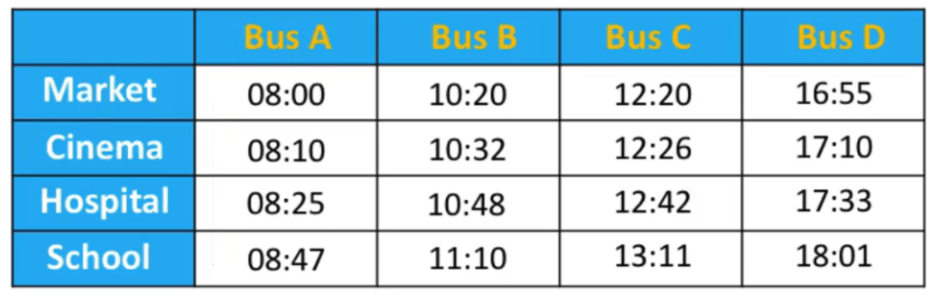error: Content is protected !!